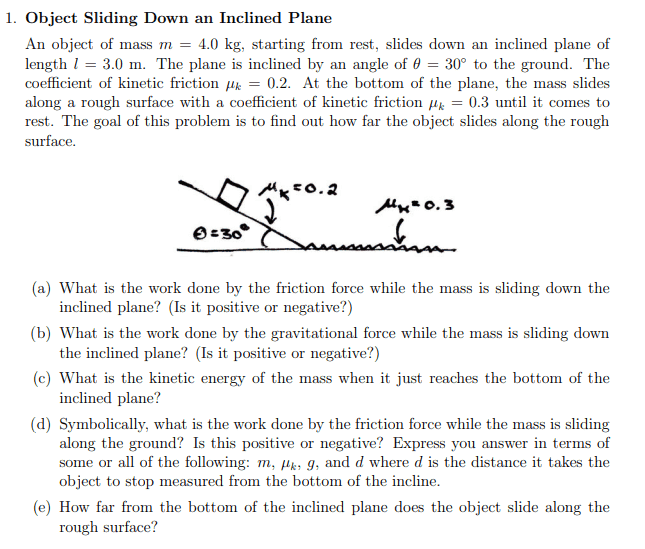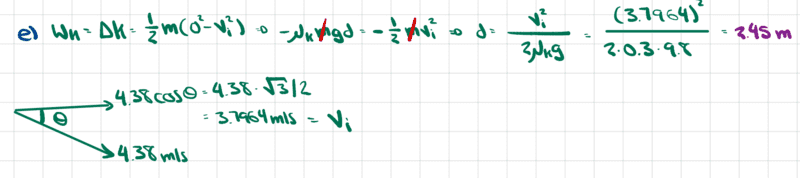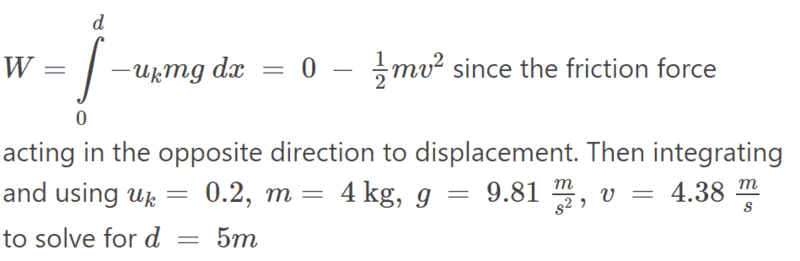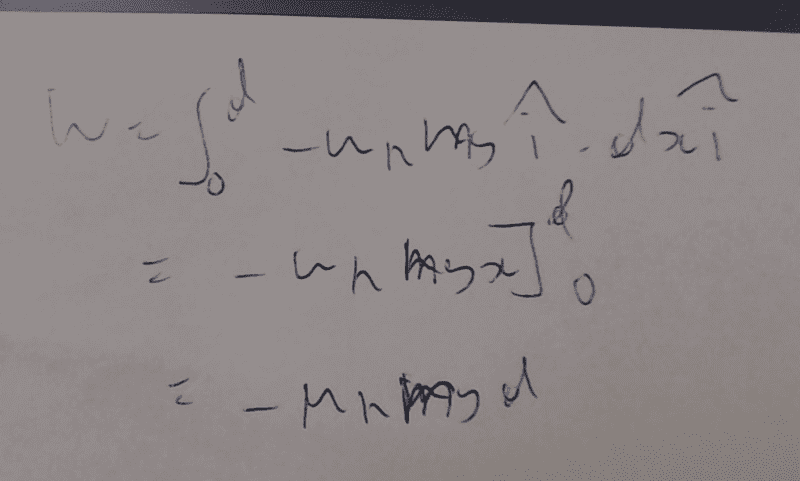# Distance a block slides along a surface with friction given with an initial velocity

ChiralSuperfields
Hi!

For this part (e) of this problem,Source: https://ocw.mit.edu/courses/8-01sc-...647ea989a352a972dc4b3dfe_MIT8_01F16_pset7.pdf

The solutions are,However, I don't understand why they only used a component of the initial velocity as it comes off the incline. I used 4.38 m/s because I thought that once the block reaches the horizontal surface, the vertical and horizontal components of the velocity from the incline would combine to give 4.38 m/s. Do you please know why or whether the solutions are wrong?

Many thanks!

Mentor
Moderator's note: Thread moved to intro physics homework forum.

Mentor
once the block reaches the horizontal surface, the vertical and horizontal components of the velocity from the incline would combine
I'm not sure what you mean by "combine". You might try thinking in terms of what property of the block is unchanged across the transition from the inclined plane to the horizontal segment.

The solutions
Where are you getting these from?

Mentor
You might try thinking in terms of what property of the block is unchanged across the transition from the inclined plane to the horizontal segment.
A hint to this is that the problem statement never asks you for the speed of the block. It does ask for something else at the transition. What?

ChiralSuperfields
I'm not sure what you mean by "combine". You might try thinking in terms of what property of the block is unchanged across the transition from the inclined plane to the horizontal segment.

Where are you getting these from?
Hi Peter,

Thank you for your replies. I got the solutions from this guy's Github - .

Many thanks,
Callum

Mentor
I got the solutions from this guy's Github
You might want to try solving the problems yourself to check whether that source is reliable. (Hint: I don't think it is.)

ChiralSuperfields
You might want to try solving the problems yourself to check whether that source is reliable. (Hint: I don't think it is.)
Hi Peter,

Yeah, I'm not sure, I did look at some of the other solutions which did seemed correct to me. The distance I calculated was 5 m which was using the velocity of the block at the bottom of the incline to be 4.38 m/s. Would you please know whether my solution is correct?

Many thanks,
Callum

Mentor
using the velocity of the block at the bottom of the incline to be 4.38 m/s
Why do you think that's correct? Not "because that's what the solution I read says". How would you do the calculation?

(Hint: you don't even need to calculate the block's velocity at all to solve the entire problem, all five parts. All you need is the fact that the work done by a force over a distance is the force times the distance.)

Mentor
(Hint: I don't think it is.)
On looking a little deeper I might have been too quick to dismiss this source as giving wrong numerical answers. However, I still don't think it works very well as a study guide.

Mentor
The distance I calculated was 5 m which was using the velocity of the block at the bottom of the incline to be 4.38 m/s.
Assuming this velocity is correct, how are you getting 5 meters?

ChiralSuperfields
Thanks for your reply. I have written up how I got 5 meters:Mentor
I have written up how I got 5 meters:
Equations in images are not permitted. Please use the PF LaTeX feature to post equations directly. You will see a "LaTeX Guide" link at the bottom left of each post window.

Mentor
I have written up how I got 5 meters
Your write up tells me nothing since you have given no equation that includes the distance ##d##. What is the equation for ##d##?

Mentor
I have written up how I got 5 meters
Note also that ##\mu_k = 0.3## for the horizontal part of the problem, not ##0.2##.

ChiralSuperfields
Your write up tells me nothing since you have given no equation that includes the distance ##d##. What is the equation for ##d##?
Thanks for your reply. I solved it the same way as the 'solutions' apart from the fact that I used the initial velocity to be 4.38 m/s. Many thanks

Homework Helper
Gold Member
2022 Award
why they only used a component of the initial velocity as it comes off the incline
The question should have made it clear exactly what happens at the bottom of the ramp.

If it is a smooth change, with a small arc connecting the two flat surfaces, at least some of the vertical component of velocity will get converted to horizontal. However, the object is shown as a block, so its moment of inertia becomes relevant. To avoid its getting quite messy, take it as a point mass at the centre of a light block.
If the radius of curvature of the arc is R its length is Rθ and the normal includes a centripetal component mu2/R, which could be a lot more than mg. This gives a frictional force μmu2/R acting over that distance and taking away μmu2θ of the KE. Note that this cannot be magicked away by making R small.

The solution you post assumes a sudden change, and that the vertical component of momentum is simply lost. This immediately takes away ##\frac 12mu^2\sin(\theta) ## of the KE. But the friction complicates matters. There will be an impulse at each end of the block, leading to impulsive friction at each, also instantly reducing the speed.

In short, a problem like this with friction is fiendishly difficult.

Last edited:
•PeroK and ChiralSuperfields
Mentor
I solved it the same way as the 'solutions' apart from the fact that I used the initial velocity to be 4.38 m/s.
If you aren't going to respond to my questions, we can just close this thread. Either you're here to get help or you're not.

As I've already said, you should not assume that the "solutions" are correct. You should solve the problem for yourself. You're not even following the problem statement since part d) of that asks you for a symbolic equation that relates ##d## to other parameters. Can you give such an equation?

ChiralSuperfields
Note also that ##\mu_k = 0.3## for the horizontal part of the problem, not ##0.2##.
Sorry I am quite bad at explaining myself. I happen to be dyslexic so apologies if I am a little slow.

Mentor
I happen to be dyslexic so apologies if I am a little slow.
It's fine to take your time. The key thing is to think through the solution for yourself, without referring to anyone else's attempt at a solution.

Mentor
The question should have made it clear exactly what happens at the bottom of the ramp.
I agree this is a key missing piece in the question. I have been assuming that there would be something in the course notes or lectures that would clarify this (since it's an introductory physics course I would not expect it to go into all of the complexities you describe), but so far I have not found anything.

ChiralSuperfields
The question should have made it clear exactly what happens at the bottom of the ramp.

If it is a smooth change, with a small arc connecting the two flat surfaces, at least some of the vertical component of velocity will get converted to horizontal. However, the object is shown as a block, so its moment of inertia becomes relevant. To avoid its getting quite messy, take it as a point mass.
If the radius of curvature of the arc is R its length is Rθ and the normal includes a centripetal component mu2/R, which could be a lot more than mg. This gives a frictional force μmu2/R acting over that distance and taking away μmu2θ of the KE. Note that this cannot be magicked away by making R small.

The solution you post assumes a sudden change, and that the vertical component of momentum is simply lost. This immediately takes away ##\frac 12mu^2\sin(\theta) ## of the KE. But the friction complicates matters. There will be an impulse at each end of the block, leading to impulsive friction at each, also instantly reducing the speed.

In short, a problem like this with friction is fiendishly difficult.
Thank you for your reply, yeah it seems a bit dodge to me that that vertical component of the momentum is suddenly loss. If I consider a ball rolling down the incline, I don't think it's vertical component would be lost like that.

Many thanks,
Callum

ChiralSuperfields
Note also that ##\mu_k = 0.3## for the horizontal part of the problem, not ##0.2##.
Yes sorry, my mistake.

ChiralSuperfields
It's fine to take your time. The key thing is to think through the solution for yourself, without referring to anyone else's attempt at a solution.
Ok thank you!

ChiralSuperfields
If you aren't going to respond to my questions, we can just close this thread. Either you're here to get help or you're not.

As I've already said, you should not assume that the "solutions" are correct. You should solve the problem for yourself. You're not even following the problem statement since part d) of that asks you for a symbolic equation that relates ##d## to other parameters. Can you give such an equation?
I agree, I cannot assume the "solutions" are correct since they have not been credited by MIT. I did solve part (d) to get the same symbolic equation as the "solutions" which I integrated the force with respect to position using the limits, zero and d.

Mentor
If I consider a ball rolling down the incline, I don't think it's vertical component would be lost like that.
A rolling ball introduces additional complexities, though, because some of the work done on the ball as it rolls down the incline goes into increasing its rate of rotation instead of its linear velocity. This is a fairly common "gotcha" for physics students analyzing experiments with inclined planes like those done by Galileo and getting stumped about the results they are seeing.

Mentor
I did solve part (d) to get the same symbolic equation as the "solutions"
What equation is that? Please post it explicitly here.

ChiralSuperfields
Ok let me just take a photo of my working.

ChiralSuperfieldsMentor
Ok let me just take a photo of my working.
Again, this is not allowed. Please use the PF LaTeX feature to post equations directly in the thread.

ChiralSuperfields
Again, this is not allowed. Please use the PF LaTeX feature to post equations directly in the thread.
Ok thanks for letting me know.

Homework Helper
Gold Member
2022 Award
Hi!

For this part (e) of this problem,
View attachment 317728
Source: https://ocw.mit.edu/courses/8-01sc-...647ea989a352a972dc4b3dfe_MIT8_01F16_pset7.pdf

The solutions are,
View attachment 317729
However, I don't understand why they only used a component of the initial velocity as it comes off the incline. I used 4.38 m/s because I thought that once the block reaches the horizontal surface, the vertical and horizontal components of the velocity from the incline would combine to give 4.38 m/s. Do you please know why or whether the solutions are wrong?

Many thanks!
In the simplest terms, when the block reaches the end of the ramp it collides with the ground.

Imagine a car or bicycle coming to the end of a ramp like that. There would be a definite vertical bounce.

And with a block it's not clear what happens when that leading edge hits the ground.

Not a great question, IMO, as clearly you need the simplifying assumption of a simple totally inelastic collision at the bottom of the ramp.

•ChiralSuperfields
ChiralSuperfields
In the simplest terms, when the block reaches the end of the ramp it collides with the ground.

Imagine a car or bicycle coming to the end of a ramp like that. There would be a definite vertical bounce.

And with a block it's not clear what happens when that leading edge hits the ground.

Not a great question, IMO, as clearly you need the simplifying assumption of a simple totally inelastic collision at the bottom of the ramp.
Thanks for your reply, I see what you mean. If there was no friction my solution would be correct I think. Many thanks!

Homework Helper
Gold Member
2022 Award
Thanks for your reply, I see what you mean. If there was no friction my solution would be correct I think. Many thanks!
If there were no friction, the block would never stop. Even if the slope and ground were pure ice, there would still be a collision between the leading edge and the horizontal ice. In practical terms, the ice would be chipped and energy lost.

Even with a ball the vertical motion would be translated into a sequence of bounces, rather than horizontal velocity.

•ChiralSuperfields
Homework Helper
Gold Member
2022 Award
Thanks for your reply, I see what you mean. If there was no friction my solution would be correct I think. Many thanks!
No, you are missing the point. The sudden loss of speed on hitting the horizontal surface in the given solution has nothing to do with friction. It is because of the inelastic impact in the vertical direction. And making it elastic doesn’t help; it would bounce, but the vertical momentum and energy it had on the ramp still does not contribute to the horizontal speed after impact.

•ChiralSuperfields and PeroK
ChiralSuperfields
If there were no friction, the block would never stop. Even if the slope and ground were pure ice, there would still be a collision between the leading edge and the horizontal ice. In practical terms, the ice would be chipped and energy lost.

Even with a ball the vertical motion would be translated into a sequence of bounces, rather than horizontal velocity.
Ok thank you!